您可以捐助，支持我们的公益事业。 1元 10元 50元 认证码：必填求知 文章 文库 Lib 视频 iPerson 课程 认证 咨询 工具 讲座 Modeler Code要资料订阅捐助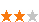886 次浏览     评价： 好 中 差
2019-2-26

 编辑推荐: 本文来自于csdn，本文是一篇机器学习Scikit-learn的笔记，主要介绍Scikit-learn的安装和使用，希望会对您的学习有所帮助。

Scikit-learn安装

sklearn库依赖于numpy、scipy、matplotlib库，首先安装numpy，然后安装scipy、matplotlib库，最后安装scikit-learn库。可以通过anaconda进行安装或者通过依赖关系，逐个进行pip install进行安装。

Scikit-learn的数据集介绍

scikit-learn数据集如下图所示，包括了小数据集和大数据集，采用函数方法调用。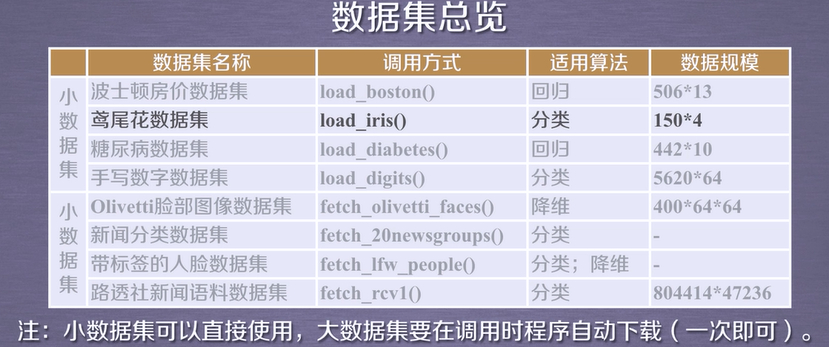# 加载boston房价信息示例 from sklearn.datasets import load_boston data, target = load_boston(return_X_y=True) print(data.shape) print(target.shape)

 #加载手写数字库 from sklearn.datasets import load_digits import matplotlib.pyplot as plt digits = load_digits() plt.matshow(digits.images) plt.show()

sklearn库包括6大部分，包括分类、回归、聚类、降维、模型选择以及数据预处理。具体的函数如下图所示：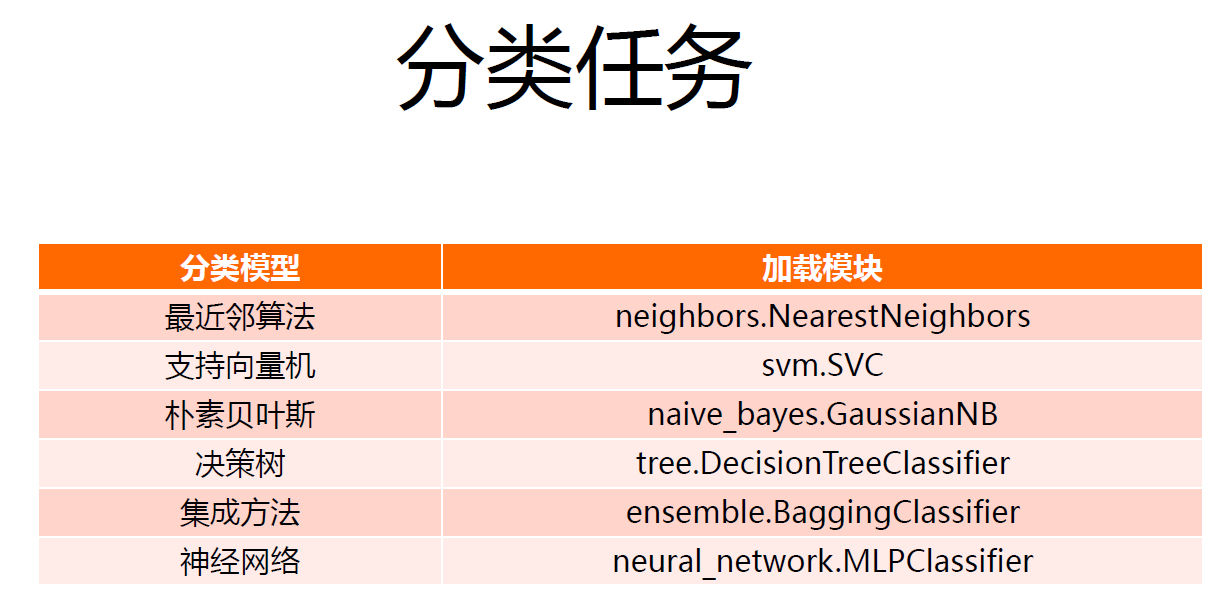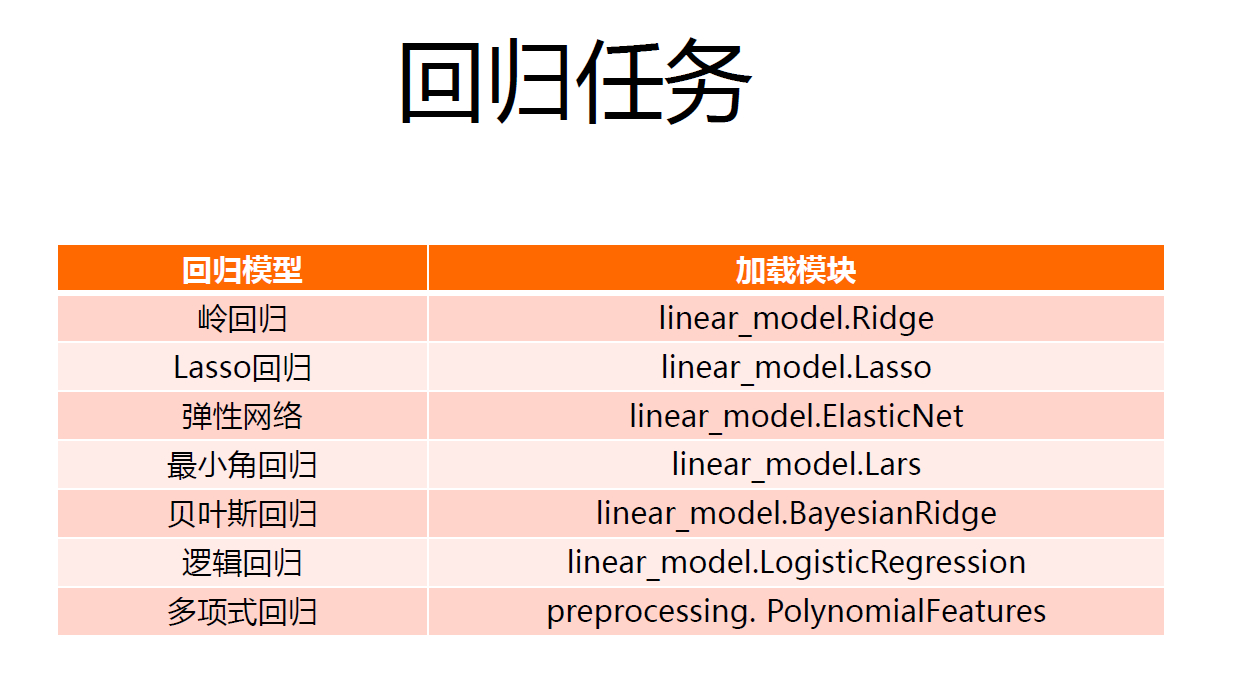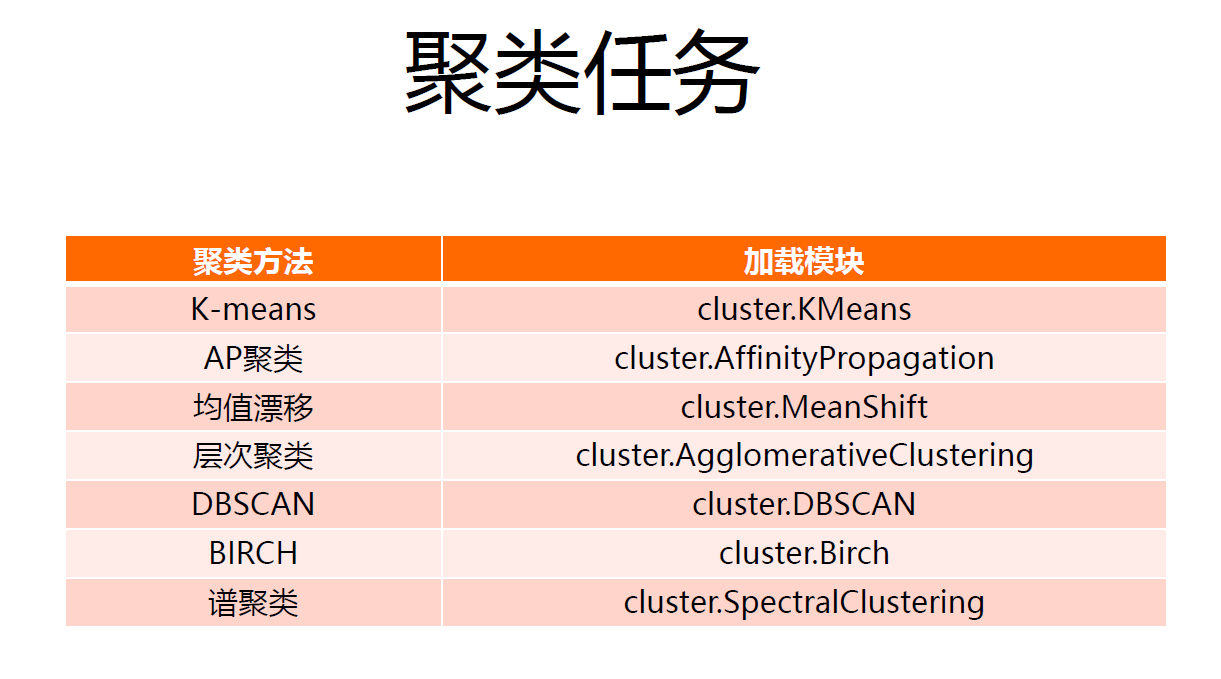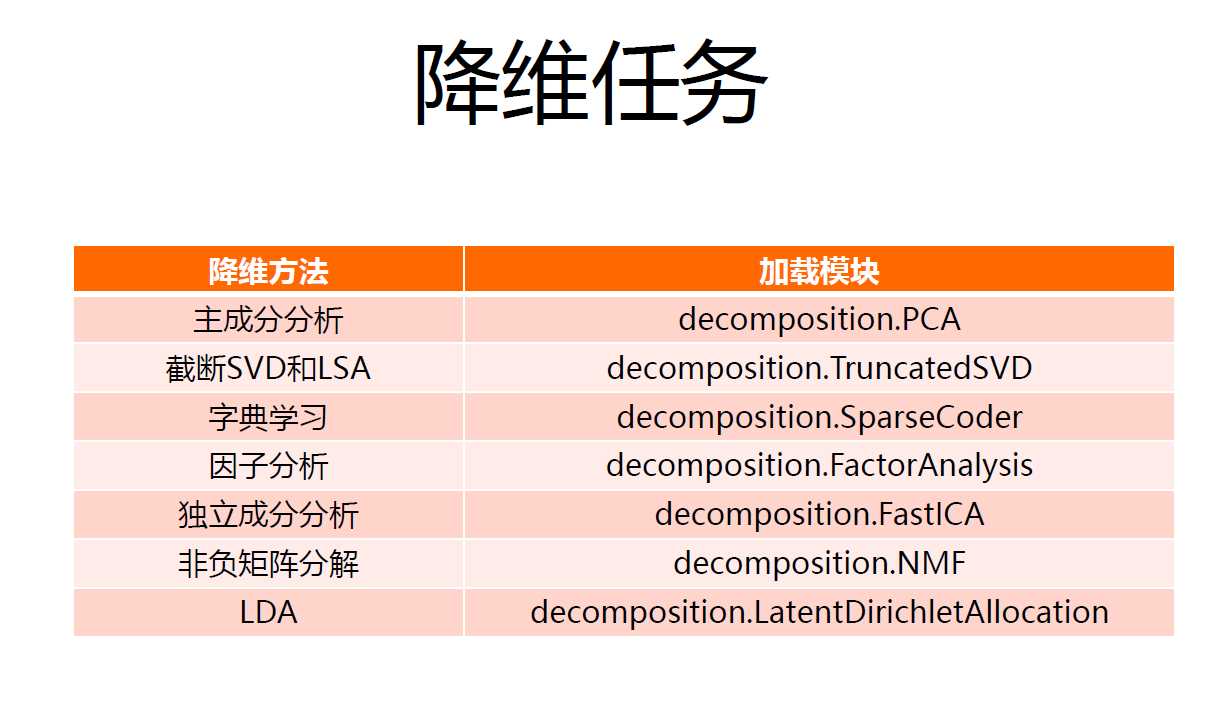Sklearn无监督学习使用

sklearn聚类算法包含于sklearn.cluster中，包含了k-means、邻近传播算法、DBSCAN等。

sklearn.cluster可以采用多种数据形式作为输入，标准形式为[样本个数 特征个数]，还可以采用其他方式进行。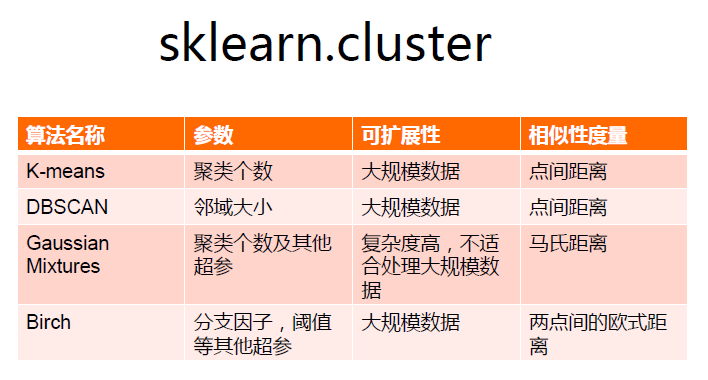sklearn降维算法包含于sklearn.decomposition，目前有7种降维算法。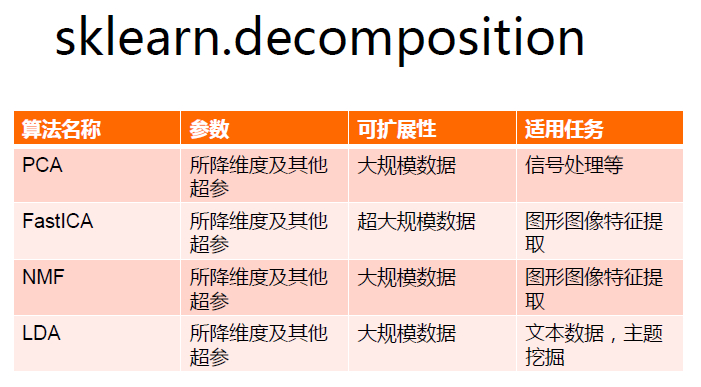kmeans算法将数据分为k个簇，簇内相似度较高，簇间相似度较低。其操作过程如下图所示。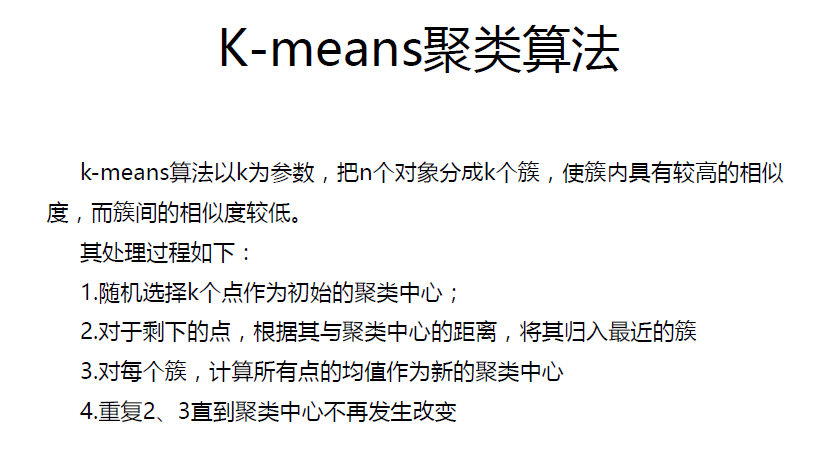from sklearn.cluster import KMeans from sklearn.datasets import load_iris if __name__ == '__main__': # import iris data: x is the iris feature and y is the class x, y = load_iris(return_X_y=True) # the number clusters is 3 km = KMeans(n_clusters=3,init='k-means++', max_iter=10000) label = km.fit_predict(x) # evaluate the accuracy error_cnt = 0 sum_cnt = 0 for i in range(len(label)): if (y[i] != label[i]): error_cnt =error_cnt + 1 sum_cnt = sum_cnt + 1 print("Error rate:%.2f" % (error_cnt/sum_cnt))

kmeans可以用于图像分割，即利用图像分灰度、颜色、纹理、形状等特征将图像分为若干不重叠区域，使这些特征在同一区域具有相似性而不同区域呈现冥想差异性。图像分割常用技术包括了阈值分割、边缘分割、直方图法和聚类分析、小波变换等。kmeans分割可以实现图像中相似特征聚类，从而完成分割情况。对于同一类采用相同颜色标记，最终可以形成分割图像。实例如下，一个较重要的包为PIL，实例代码如下：

 import PIL.Image as image def loadData(filePath): f = open(filePath,'rb') data = [] img = image.open(f) m,n = img.size for i in range(m): for j in range(n): x,y,z = img.getpixel((i,j)) data.append([x/256.0,y/256.0,z/256.0]) f.close() return np.mat(data),m,n

DBSCAN基于密度进行聚类，不需要指定聚类个数。DBSCAN算法将数据点分为三类：核心点、边界点和噪音点，这依据一点的邻域eps内其他点个数的情况是否大于minpts来决定。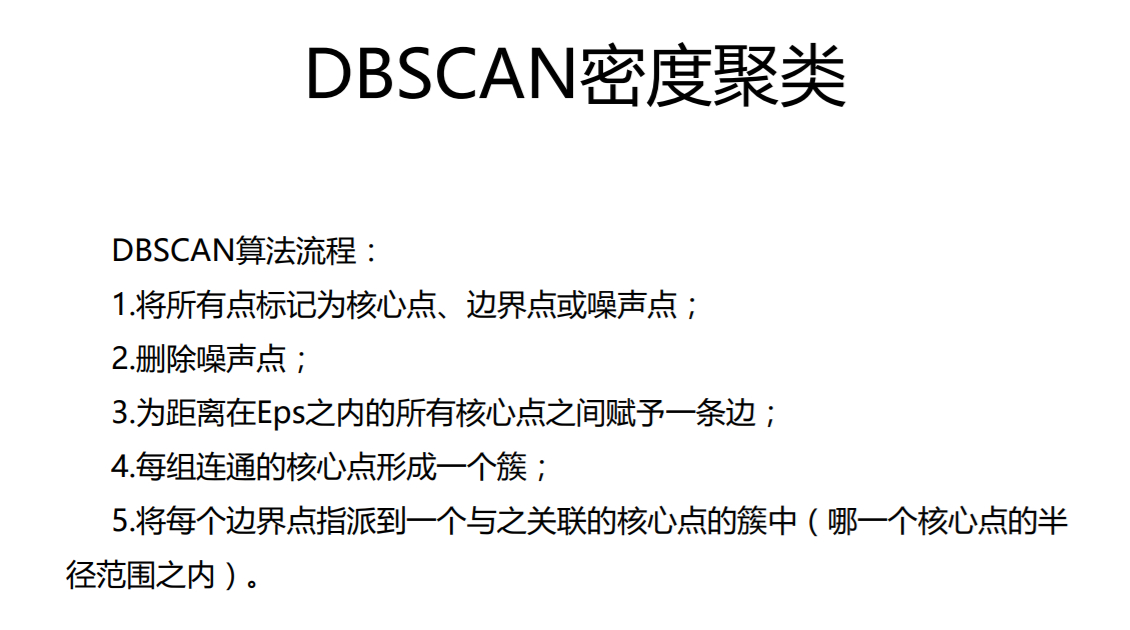# 统计每一类别元素个数的代码 for i in range(0,nc): numberOfClass.append(len(label[label[:] == i])) print(numberOfClass)

 # 统计分类个数的代码 n_clusters = len(set(labels))-(1 if -1 in labels else 0)

 # DBSCAN核心代码 db = DBSCAN(eps=1,min_samples=20).fit(x) labels = db.labels_

DBSCAN基于密度分布进行聚类，对于iris数据难以实现有效聚类。

PCA，主成分分析，较为常用的一种降维方法，可以用于高维数据的探索和可视化，还可以用作数据压缩和预处理。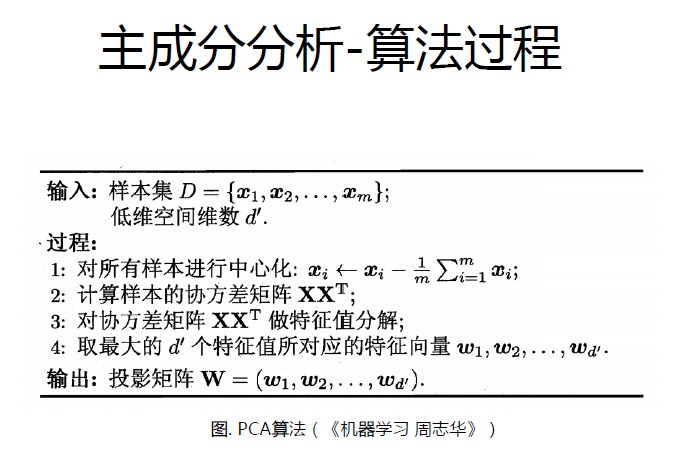# 原数据为四维的，使用PCA对数据进行降维， # 实现在二维平面的可视化 import matplotlib.pyplot as plt from sklearn.decomposition import PCA from sklearn.datasets import load_iris # 加载数据集导入函数 data = load_iris() # 以字典形式加载数据集 y = data.target # y表示数据集中的标签 X = data.data # x表示数据集中的属性数据 pca = PCA(n_components=2) # 加载pca算法，主成分数目为2 reduced_X = pca.fit_transform(X) # 对原始数据进行降维，保存在reduced_X中 red_x, red_y = [], [] blue_x, blue_y = [], [] green_x, green_y = [], [] # 按类别将降维后的数据进行保存 for i in range(len(reduced_X)): # range(5) #代表从0到5(不包含5) if y[i] == 0: red_x.append(reduced_X[i]) red_y.append(reduced_X[i]) elif y[i] == 1: blue_x.append(reduced_X[i]) blue_y.append(reduced_X[i]) else: green_x.append(reduced_X[i]) green_y.append(reduced_X[i]) # 按照鸢尾花的类别将降维后的数据点保存在不同的列表中 plt.scatter(red_x, red_y, c='r', marker='x') # 画x关于y的散点图，c：color，r：red。 # maker：标记点的形状。x：x形；D：钻石形；.：点形 plt.scatter(blue_x, blue_y, c='b', marker='D') plt.scatter(green_x, green_y, c='g', marker='.') plt.show()

NMF，非负矩阵分解，是在矩阵所有元素为非负数这一条件约束情况下的矩阵分解方法。其思想为，对于一个非负矩阵，可以找到另外的矩阵W和H，这两者也是非负矩阵，使得W乘以H接近矩阵V。其中W矩阵称为基础图像矩阵，而H矩阵为系数矩阵。W矩阵类似于V矩阵中抽取的特征。

NMF的算法需要进一步进行探究，可以使用NMF方法进行，关键参数有n_components，init表示W和H矩阵的初始化方法。

NMF和PCA可以直接替代使用，二者参数接近。

Sklearn监督学习使用

sklearn库中分类算法未被统一封装，因此其import方式各有不同，其分类算法包括了KNN，朴素贝叶斯，SVM，决策树，神经网络模型等等，既有线性分类器，也有非线性分类器。

sklearn库的回归函数封装在linear_model和prepocessing中，其中线性回归函数包括线性回归、岭回归、LASSO回归，非线性回归如多项式回归等。

KNN的基本思想是通过计算待分类数据与训练集数据的距离，取前K个点，将待分类数据划分到出现次数最多的那个类别。

sklearn中采用sklearn.neighbors.KNeighborsClassifier创建K近邻分类器，关键参数包括n_neighbors和weights。为了能够对于大数据进行处理，可以采用合适的计算临近点的方法。实际使用过程中，倾向于使用较小的K，并且使用交叉验证得到最优的K值。

 # KNN简易代码 from sklearn.neighbors import KNeighborsClassifier x = [,,,] y = [0,0,1,1] neigh = KNeighborsClassifier(n_neighbors=3) neigh.fit(x,y) print(neigh.predict([[1.1]]))

sklearn可以使用sklearn.tree.DecisionTreeClassifier进行分类，参数有criterion，可以选择gini或者entropy；max_features可以选择决策树节点进行分类时，从多少个特征中选取最优特征。

 from sklearn.datasets import load_iris from sklearn.tree import DecisionTreeClassifier from sklearn.model_selection import cross_validate # 默认使用gini分类，这里采用entropy分类 clf = DecisionTreeClassifier(criterion='entropy') iris = load_iris() print(cross_validate(clf,iris.data,iris.target,cv=10))

 # 朴素贝叶斯示例代码 import numpy as np from sklearn.naive_bayes import GaussianNB X = np.array([[-1,-1],[-2,-1],[-3,-2],[1,1],[2,1],[3,2]]) Y = np.array([1,1,1,2,2,2]) clf = GaussianNB(priors=None) clf.fit(X,Y) print(clf.predict([[-0.8,-1]]))

SVM分类方法在sklearn.svm.SVC中，代码如下所示，需要设置kernel参数，例如rbf，linear,poly,sigmoid等。同时针对本分类，可以产生训练集和测试集。

 from sklearn.datasets import load_iris from sklearn.model_selection import cross_validate from sklearn import svm clf = svm.SVC(kernel='rbf') x,y = load_iris(return_X_y=True) print(cross_validate(clf,x,y,cv=10))

MLP，即多层全连接神经网络。对于DBRHD数据集，其大小为32*32的文本矩阵，而MLP采用向量输入，因此需要展开文本矩阵，输出采用one-hot vectors。Hidden layer的层数和神经元个数均会影响识别准确率。

 clf = MLPClassifier(hidden_layer_sizes=(100,), activation='logistic', solver='adam', learning_rate_init = 0.0001, max_iter=2000) print(clf) clf.fit(train_dataSet,train_hwLabels) #read testing dataSet dataSet,hwLabels = readDataSet('testDigits') res = clf.predict(dataSet) #对测试集进行预测

sklearn提供了数据预处理模块Imputer，自动生成训练集和测试集的模块train_test_split，预测结果评估模块classification_report。注意，train_test_split已经被删除。

 from sklearn.preprocessing import Imputer from sklearn.cross_validation import train_test_split from sklearn.metrics import classification_report

 df = pd.read_table(file, delimiter=',', na_values='?', header=None) imp = Imputer(missing_values='NaN', strategy='mean', axis=0) imp.fit(df) df = imp.transform(df) feature = np.concatenate((feature, df))

train_test_split函数可以将数据顺序打乱，而classification_report可以生成数据分类的结果准确度。

 print(classification_report(y_label, y_clf_result))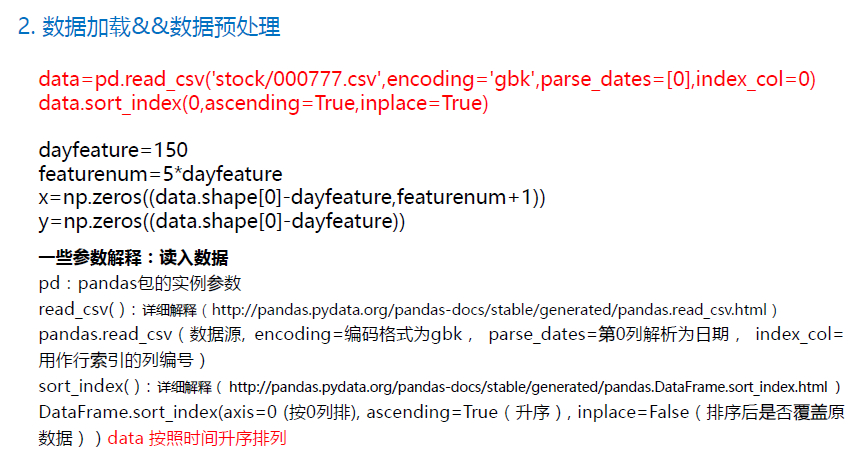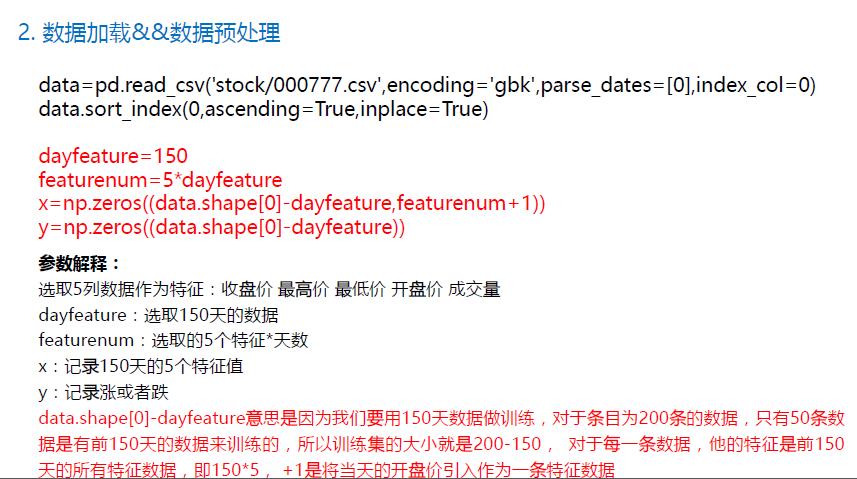from sklearn.prepocessing import PolynomialFeatures poly_reg = PolynomialFeatures(degree = 2) X_poly = poly_reg.fit_transform(datasets_X) lin_reg_2 = linear_model.LinearRegression() lin_reg_2.fit(X_poly, datasets_Y)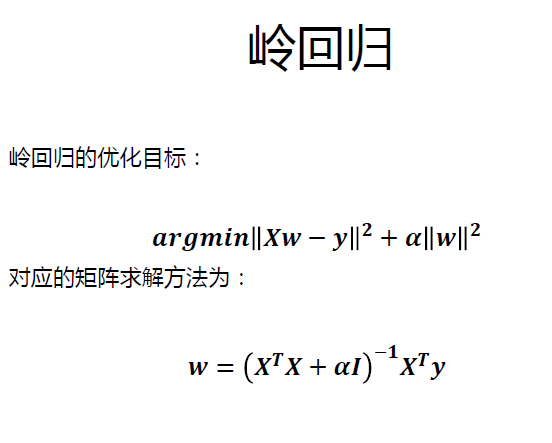DQN: 利用深度网络，可以实现深度强化学习，得到较好的学习效果。该方法直接学习环境和动作状态函数的映射关系，得到问题的解。886 次浏览     评价： 好 中 差订阅捐助
 相关文章 我们该如何设计数据库 数据库设计经验谈 数据库设计过程 数据库编程总结
 相关文档 数据库性能调优技巧 数据库性能调整 数据库性能优化讲座 数据库系统性能调优系列